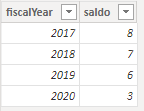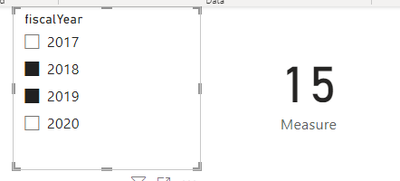cancel
Showing results for
Did you mean:Helper I

## Dax calculation

Hello guys I need these  calculation to work for a card visual to display the value of sum auditfile

CALCULATE(SUM('2 (Auditfile)'[saldo]), IF(VALUES('2 (Auditfile)'[fiscalYear])>2018,[fiscalyear]-1))

why doesnt it work i want the value of sum saldo
many thanks :):):)
6 REPLIES 6Helper I

i need something like this :
if(selectedvalue(auditfile[fiscalyear]=2019,selectvalue(auditfile[fiscalyear]-1),
calculate(sum(auditfile[saldo])

but this doesnt work, so i need a measure that if i select a fiscal year in slicer it gives me the sum of saldo of the previous year but i cant use the previous year functie because the way i have the dataMemorable Member

My understanding is that you want sum of saldo when for dates after the fiscal year 2018. Try:

``CALCULATE(SUM('2 (Auditfile)'[saldo]),FILTER('2 (Auditfile)','2 (Auditfile)'[fiscalYear])>2018))``

Works for you? Mark this post as a solution if it does!
Consider taking a look at my blog: How to Export Telemetry Data from Azure IoT Central into Power BIHelper I

hey @Shaurya  you got it almost i want sum of saldo when in a slicer is 2018 selected i want the sum saldo of 2017, when in the slicer 2019 is selected i want the sum of saldo from 2018, when 2020 is selected i want sum of saldo from 2019 etc etc       previeus year function doesnt work for me the way my data is but it think its something like your dax formulaCommunity Support

Here I suggest you to create an unrelated Fiscal Year Table for slicer and create a measure to achieve your goal.

My Sample:Fiscal Year Table:

``Fiscal Year = VALUES(Auditfile[fiscalYear])``

Measure:

``````Measure =
VAR _SELECTYEAR = VALUES('Fiscal Year'[fiscalYear])
VAR _PREVIOUSYEAR = SUMMARIZE( ADDCOLUMNS(_SELECTYEAR,"PY",[fiscalYear] - 1),[PY])
RETURN
CALCULATE(SUM(Auditfile[saldo]),FILTER(Auditfile,Auditfile[fiscalYear] IN _PREVIOUSYEAR))``````

Result is as below.Best Regards,
Rico Zhou

If this post helps, then please consider Accept it as the solution to help the other members find it more quickly.Helper I

i need something like this but this doesnt work:
if(selectedvalue(auditfile[fiscalyear])>=2019, selectvalue(auditfile[fiscalyear] -1),
calculate(sum(auditfile[saldo])

so if i select a year in  a slicer i want the value of the previous year. the previous year functie does not work for me the way i have the data.Helper I

hey @v-rzhou-msft if i use your meassure it returns blanks if i put the new fiscalyear table in it if i use the slicer with auditfile fiscalyear it returns the same value of the year i selected but i want the sum of saldo 2018 if i select 2019 in the slicer iwant the sum of saldo 2019 if i select 2020 enz enz